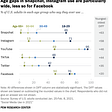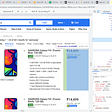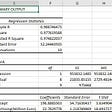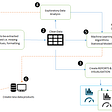# Data Preprocessing Phase

`df_na = (df.isnull().sum() / len(df)) * 100df_na = df_na.drop(df_na[df_na == 0].index).sort_values(ascending=False)[:100]missing_data = pd.DataFrame({'Missing Ratio' :df_na})missing_data.head(50)`
`missingdataplot=pd.DataFrame(missing_data)missingdataplot.plot(kind='barh', figsize=(12, 14),  zorder=2, width=0.85)plt.suptitle('Missing Value Ratio',fontsize=20)txt="Figure 3 - Missing Value Ratio of Features after dropping"plt.figtext(0.5, 0.05, txt, wrap=True, horizontalalignment='center', fontsize=12)`
`mask = df.isna().sum() / len(df) < 0.4reduced_df = df.loc[:, mask]print(df.shape)print(reduced_df.shape)`
`plt.figure(figsize=(16, 6))mask = np.triu(np.ones_like(df.corr(), dtype=np.bool))heatmap = sns.heatmap(df.corr(), mask=mask, vmin=-1, vmax=1, annot=True, cmap='BrBG')heatmap.set_title('Triangle Correlation Heatmap', fontdict={'fontsize':18}, pad=16);`
`corr_matrix = reduced_df.corr().abs()mask = np.triu(np.ones_like(corr_matrix, dtype=bool))tri_df = corr_matrix.mask(mask)#Correlation Value(r > 0.70)to_drop = [c for c in tri_df.columns if any(tri_df[c] > 0.70)]# Dropreduced_df1 = reduced_df.drop(to_drop, axis=1)print("The reduced_df dataframe has {} columns".format(reduced_df.shape))print("The reduced_df1 dataframe has {} columns".format(reduced_df1.shape))`

# Modeling Phase

`from sklearn.model_selection import train_test_splity = reduced_df['TARGET']X=reduced_df.drop('MODELTARGET',axis=1)`
`import lightgbm as lgbX_train, X_test, y_train, y_test = train_test_split(X, y,stratify=y, test_size=0.20, random_state=0)lgb=lgb.LGBMClassifier()lgb.fit(X_train, y_train)`

# Results & Evaluation

`from sklearn.metrics import classification_reportreport = classification_report(y_test, predicted)print(report)`
`importances = lgb.feature_importances_# Sort importancessorted_index = np.argsort(importances)# Create labelslabels = X.columns[sorted_index]# Clear current plotplt.clf()# Create plotplt.figure(figsize=(25,25))plt.barh(range(X.shape), importances[sorted_index], tick_label=labels)plt.suptitle('Predictor Importance',fontsize=20)txt="Figure 9 - Feature Importance for Light GBM Model"plt.figtext(0.5, 0.05, txt, wrap=True, horizontalalignment='center', fontsize=12)plt.show()`

# KEY TAKEAWAYS

--

--

--

## More from Buğra Balantekin

CRM Analyst — Machine Learning/Data Science

Love podcasts or audiobooks? Learn on the go with our new app.

## What Is My Home Worth? What Every Homeowner and Seller Should Know## Research Report and Qualitative vs. Quantitative## DataHub 2021 in Review## The winner takes it all## AIM: Perform data collection by web scrapping with python.## What are the Missing Values??## How to Conduct Simple Regression Analysis on Excel## Separation of Concerns : Data Pre-processing and Visualization## Buğra Balantekin

CRM Analyst — Machine Learning/Data Science

## Machine Learning & Statistical Modeling in CRM (All in One❗)(EN)